Expert Modelling: Hands-on help from Rocscience Engineers. Register Here

# Joint Shear Strength

The shear strength of the joint planes in SWedge can be modeled using any of the following strength criteria:

To define joint shear strength:

1. Select Input Data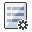from the toolbar or the Analysis menu.
2. Select the appropriate tab(s) in the Input Data dialog, choose the Shear Strength Model (Mohr-Coulomb, Barton-Bandis, Power Curve) and enter the joint strength parameters.
• For a Deterministic Analysis, define the shear strength for Joint 1, Joint 2 and Basal Joint (if defined).
• For a Probabilistic Analysis, it is possible to define statistical variability of the joint strength properties. See the Probabilistic Joint Strength topic for more information.
• For a Combination Analysis, you can define the same strength for all joints, or you can define joint strength for each joint set independently. See the Joint Strength (Combination Analysis) topic for more information.

The shear strength models are described below.

NOTE: For any of these strength models, a waviness angle can also be defined, which will have the effect of increasing the shear strength. See the Waviness Angle topic for details. If water pressure is included, then all parameters should be considered effective stress parameters. See the Water Pressure topic for details about including water pressure in the analysis.

## Mohr-Coulomb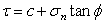Eqn.1

whereis the friction angle of the joint plane and c is the cohesion.

## Barton-Bandis

The original Barton equation for the shear strength of a rock joint is given by Eqn.2:Eqn.2

whereis the basic friction angle of the failure surface, JRC is the joint roughness coefficient, and JCS is the joint wall compressive strength [Barton, 1973, 1976]. On the basis of direct shear test results for 130 samples of variably weathered rock joints, this was later revised to Eqn.3: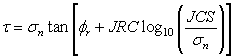Eqn.3

where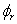is the residual friction angle of the failure surface [Barton and Choubey, 1977]. Barton and Choubey suggest thatcan be estimated from Eqn.4: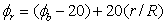Eqn.4

where r is the Schmidt hammer rebound number on wet and weathered fracture surfaces and R is the Schmidt rebound number on dry unweathered sawn surfaces. Equations 3 and 4 have become part of the Barton-Bandis criterion for rock joint strength and deformability [Barton and Bandis, 1990].

For further information on the shear strength of discontinuities, including a discussion of the Barton-Bandis failure criterion parameters, see Practical Rock Engineering (Chapter 4: Shear Strength of Discontinuities) on the Rocscience website.

## Power Curve

The Power Curve model for shear-strength [Miller, 1988], can be expressed as:Eqn.5

where a, b and c are parameters typically obtained from a least-squares regression fit of data obtained from small-scale shear tests. The d parameter represents the tensile strength. If included, it must be entered as a positive value.